# SSC CGL Tier 2 2020 Quant Questions

The Staff Selection Commission Combined Graduation Level Examination for tier 2 was successfully conducted today. The aspirants after the examination must be keenly waiting for the analysis and to get an overview of the exam that they attempted. In this article, we will be covering the Quantitative Ability section in today’s SSC CGL examination.

SSC CGL Cut Off Comparison with Previous Years

## Quantitative Ability Section

Let us first have a look at today’s (15th November 2020) quantitative ability test in SSC CGL. The quantitative ability section consisted of 100 questions for a total of 200 marks. The candidates were allotted a total time of two hours to attempt this test. Our experts after having a conversation with the aspirants who appeared in today’s exam and after getting their analysis came up with the below questions that were asked in today’s SSC CGL 2020 Exam.

SSC CGL Tier-2 Previous Year Exam Analysis

Q. The area of a cuboid’s three faces is 3,12,16. Find the volume of cuboid?
Q. 27(x+y)^3 -8(x-y)^3 = (x+5y)(Ax^2+By^2+Cxy) , find A+B+C =?
Q. 3x^2-5x+1=0 , then X^2+ 1/9x^2 = ?
Q. If from 9, 11,15 & 21, x is subtracted then the resultant numbers are in same proportion, then find the mean proportion of (3x-2) & (8x+1)
Q. A tank can be filled by A in 43.2 minutes and b can fill it in 108 minutes and C empties 3 liters/minute.If all three can fill it in 54 minutes then find the capacity of the tank?
Q. If 525ABC is divisible by 7 , 11 & 13 ,then Find 15A^2+B^2+C?
Q. A money is distributed among A B C in ration 3 : 4 : 5 , but by mistake it is distributed in the ratio of 1/3:1/4:1/5. Because of this a got 1188 Rs more find the total money?
Q. If a+b+c= 6 and a^3+ b^3+c^3 -3abc = 372 find ab+bc+ca=?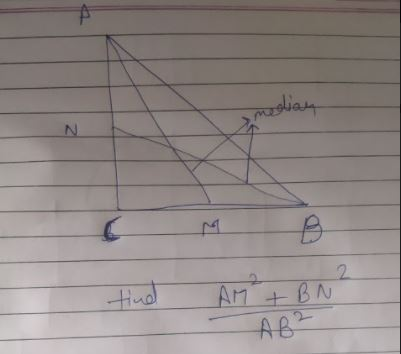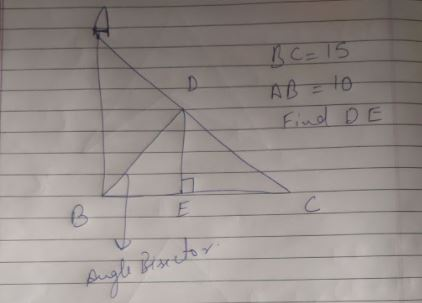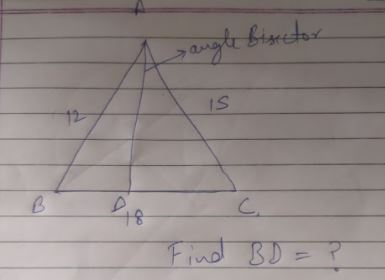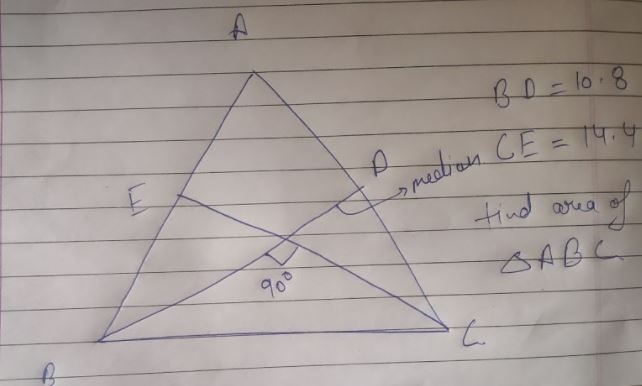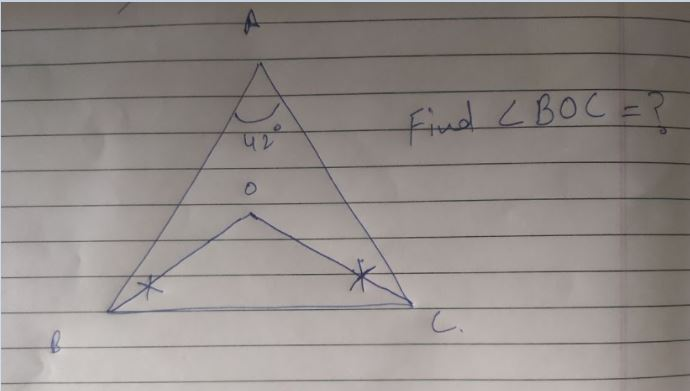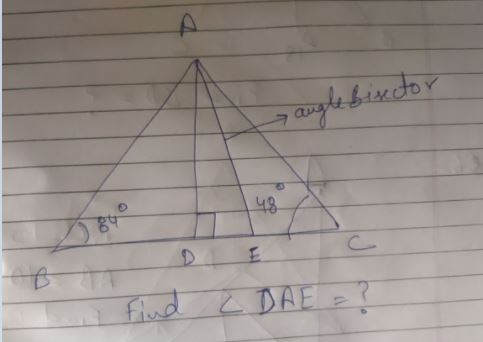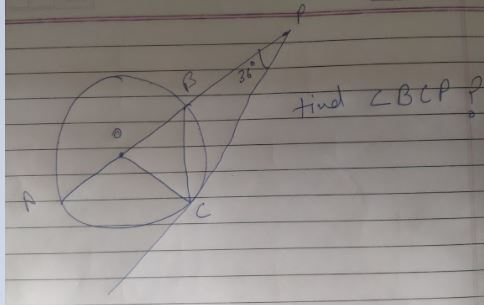Q1. AB is diameter of a circle with centre ‘O’ and ABCD is a cyclic quadrilateral, ∠COD = 60°, On extending AD and BC they meet at P. Find ∠APB.
(a) 30°
(b) 45°
(c) 60°
(d) 80°

Q2. In the adjoining figure CD is perpendicular on AB. CN is the angle bisector of ∠ACB. ∠CAN = 50°, then the angle NCD is :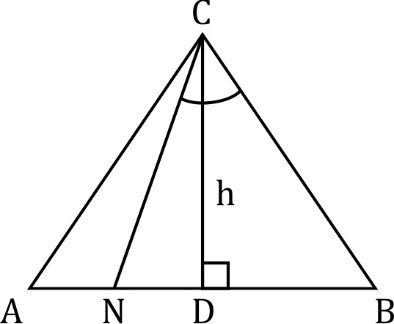(a) 1/3∠ACB
(b) ∠CAB-∠CBA
(c)1/2(∠CBA~∠BAC)
(d) can’t be determined

Q3. ABC is a triangle in which ∠CAB = 80° and ∠ABC = 50°, AE, BF and CD are the altitudes and O is the orthocenter. What is the value of ∠AOB ?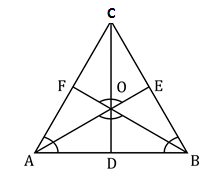(a) 65°
(b) 70°
(c) 50°
(d) 130°

Q4. ABC is a right angle triangle ∠B = 90° and BD is the median. O is the centroid of triangle. Find the length of OB if AB = 9 cm and AC = 41 cm.
(a) 41/3 cm
(b) 41/2 cm
(c) 41/4 cm
(d) 41/6 cm

Q5. ∆ABC is drawn to circumscribe a circle of radius 4 cm such that the segment BD and DC into which BC is devided by point of contact D are of length 8 cm and 6 cm respectively.Find sides AB and AC?
(a)16 cm and 14 cm
(b)15cm and 13cm
(c)13cm and 12 cm
(d)none of these

Q6. P is a point outside a circle and is 13 cm away from its centre a secant is drawn from point P intersect circle at A and B. PA = 9 cm and AB = 7 cm the radius of the circle is ?
(a) 10 cm
(b) 7 cm
(c) 8 cm
(d) 5 cm

Q7. Find radius of smaller circle with centre C3.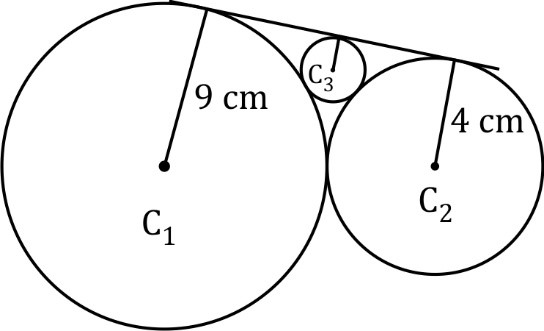(a) 2.88 cm
(b) 1.36 cm
(c) 1.44 cm
(d) 2.12 cm

Q8. In the given diagram CT is tangent at C, making an angle of π/4 with CD. O is the centre of the circle. CD = 10 cm. What is the perimeter of the shaded region (∆AOC) approximately ?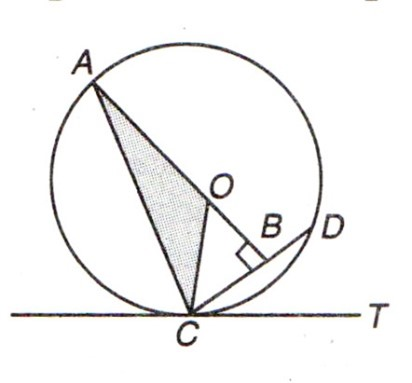(a) 27 cm
(b) 30 cm
(c) 25 cm
(d) 31 cm

Q9. A smaller circle touches internally to a larger circle at A and passes through the centre of the larger circle. O is the centre of the larger circle and BA, OA are the diameters of the larger and smaller circles respectively. Chord AC intersects the smaller circle at a point D. If AC = 12 cm, then AD is :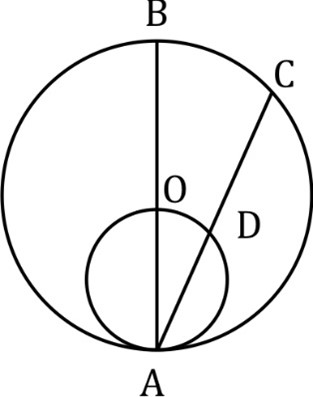(a) 4 cm
(b) 6 cm
(c) 5.6 cm
(d) data insufficient

Q10. In the given figure ADEC is a cyclic quadrilateral, CE and AD are extended to meet at B. ∠CAD = 60° and ∠CBA = 30°. Given that BD = 6cm and CE = 5√(3 )cm. What is the ratio of AC : AD ?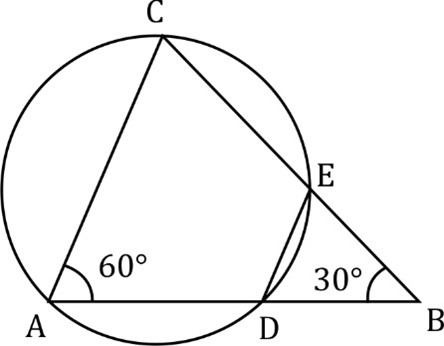(a) 3/4
(b) 4/5
(c) (2√3)/5
(d) can’t be determined

Q11. In a right angle triangle ABC, ∠A is right angle DE is parallel to the hypotenuse BC and the length of DE is 65% the length of BC, what is the area of ∆ADE, If the area of ∆ABC is 68 m^2 ?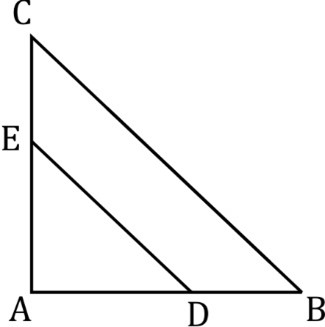(a) 27.83 cm^2
(b) 41.6 cm^2
(c) 28.73 cm^2
(d) None of the above

Q12. In the figure given below, AB is the chord of a circle with centre O. AB is extended to C such that BC = OB. The straight line CO is produced to meet the circle at D. If ∠ACD = y degrees and ∠AOD = x degrees such that x=ky, then the value of k is: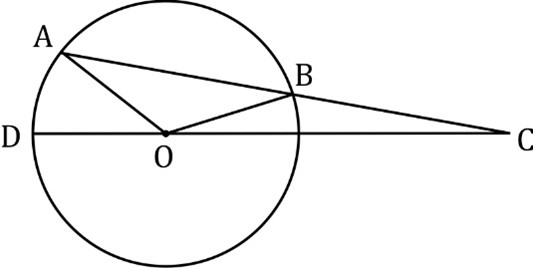(a) 3
(b) 2
(c) 1
(d) None of these

Q13. Consider the triangle ABC shown in the following figure where BC = 12 cm, DB = 9 cm, CD = 6 cm and ∠BCD = ∠BAC.What is the ratio of the area of triangle ADC to that of the triangle BDC ?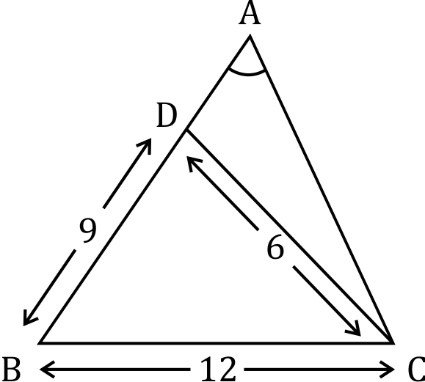(a) 7/9
(b) 8/9
(c) 6/9
(d) 5/9

Q14. A semi-circle is drawn with AB as its diameter. From C, a point on AB, a line perpendicular to AB is drawn meeting the circumference of the semi-circle at D. Given that AC = 2 cm and CD = 6 cm, the area of the semi-circle (in sq cm) will be :
(a) 32 π
(b) 50 π
(c) 40.5 π
(d) 81 π

Q15. If a+b+c=p,abc=q and ab+bc+ca=0, then what is the value of a^2 b^2+b^2 c^2+c^2 a^2 ?
(a) 2pq
(b)-2pq
(c) 3pq
(d) -3pq

Q16. A large solid sphere is melted and moulded to form identical right circular cones with base radius and height same as the radius of the sphere. One of these cones is melted and moulded to form a smaller solid sphere. Then the ratio of the surface area of the smaller to the surface area of the larger sphere is
(a) 1∶3^(4/3)
(b) 1∶2^(3/2)
(c) 1∶3^(2/3)
(d) 1∶2^(4/3)

Q17. If α is an acute angle and 2 sin⁡α+15 cos^2⁡α=7, then the value of cot α is
(a) 4/3
(b) 5/4
(c) 4/5
(d) 3/4

Q18. If θ is a positive acute angle and tan 2θ tan 3θ = 1, then the value of (2 cos^2⁡〖5θ/2〗-1) is
(a) -1/2
(b) 1
(c) 0
(d) 1/2

Q19. If (a + b)² = 21 + c², (b + c)² = 32 + a² and (c +a)² = 28 + b², find a + b +c = ?
(a) 5
(b) 9
(c) 3
(d) 1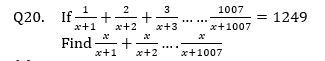(a) 142
(b) 242
(c) -242
(d) 224

S1.Ans(c)
Sol.

OD = OC = radius of circle
∴ ∠ODC= ∠OCD=60°
∠OBC= ∠OCB=y (say)
∴ ∠ADC + ∠ABC = 180°
60+x+y=180°
x+y=120°
∠APB=180-(x+y)
∠APB = 60°

S2.Ans(c)
Sol.
∠NCD =1/2 (∠CAB ~∠CBA)

S3.Ans(d)
Sol.

∠CEO = ∠CFO = 90°
∠ECF = 180 – (80° + 50°)
∠ECF = 50°
∠EOF = 360° – (90° + 90° + 50°)
∠EOF = 130°
∠AOB = ∠EOF = 130°

S4.Ans(a)
Sol.

D is mid point of AC
BD = BO + OD
3/2 BO=BD (∵ BO = 20D)
BO = 2/3×41/2
BO = 41/3 cm

S5. Ans(b)
Sol.

BE = BD = 8 cm
CD = CD = 6 cm
And
AE = AF = x (say)
Area of ∆ABC = area of ∆BOC + area of ∆AOC + area of ∆AOB
Area of ∆ ABC = 1/2×4×14+1/2 (8+x)×4+1/2 (x+6)×4
Area of ∆ABC = 4(14+x)
S = (14+(x+6)+(x+8))/2
S = (14+x)
Area of ∆ABC = √(S(S-a)(S-b)(S-c) )
=√((14+x)(14+x-14)(14+x-x-6)(14+x-x-8) )
4(14+x)=√((14+x)x×8×6)
16(14+x)^2=48(14+x)x
3x=14+x
x=7
∴ AB = 15 cm, AC = 13 cm

S6.Ans(d)
Sol.

Let centre of circle is ‘O’ and
PC = 13 – r, PD = 13 + r
PC × PD = PA × PB
⇒(13-r)(13+r)=9×16
⇒r=5 cm

S7.Ans(c)
Sol.
Common tangent of two touching circle = √4Rr
(where R and r radius of both circle)
√(4R_1 R_2 )=√(4R_1 R_3 )+√(4R_2 R_3 )
∴√(4×9×4)=√(4×9×R_3 )+√(4×R_3×4)
12=√(R_3 ) [6+4]
R_3=1.44 cm

S8.Ans(a)
Sol.
∠TCD=π/4=45°
∴ ∠OCB = 45° = ∠BOC
OB = BC = CD/2=5 cm
Radius of circle = OC =5√2
AC=√(AB^(2 )+ BC^2 )
AC=√((5√2+5)^2+5^2 )=√(50+25+50√2+ 25)
AC=√(100+50√2)
AC=5 √(4+2√2)
Required perimeter =AC+OC+OA
=5√(4+2√2) +5√2+ 5√2
=10√2+ 5√(4+2√2)
≈27.20

S9.Ans(b)
Sol.
AO = OB (Radius of larger circle)

S10.Ans(a)
Sol.
∴ ∠DEC = 120° (∵ ACED is a cyclic quadrilateral)
∠DEB = 60°
∴ ∠BDE = 90°
⇒ ∆BDE is a right angle triangle
BD = 6 cm
We can get, BE =4√3,and DE=2√3
∠ACB = 90° (∵ ∠ADE = 90°)
∴ ∆ ABC is a right angle triangle
BC = CE + BE
BC = 5√3+ 4√3
BC=9√3 cm
∠CAB = 60° and ∆ABC is a right angle triangle we can get
AC = 9, AB = 18

S11.Ans(c)
Sol.
DE/BC=65/100
DE/BC=13/20
(Area of ∆ADE)/(Area of ∆ABC)=(DE^2)/(BC^2 )=169/400

S12.Ans(a)
Sol.
BC = OB (Given)
∴ ∠BOC = ∠BCO =x°
∠ABO = ∠BOC + ∠BCO
∠ABO = 2x°
∠ABO = ∠OAB = 2x°
∴ ∠AOB=180°-(∠OAB+∠ABO) (∵ OAB is a triangle)
∠AOB = 180° – 4x
∠AOD = 180° – (∠AOB + ∠BOC)
∠AOD = y=180°-(180-4x+x)
⇒y=3x
Hence k=3

S13.Ans(a)
Sol.
∆BDC ~ ∆BCA
BC/AB=BD/BC
12/AB=9/12
AB=144/9
AB = 16 cm
AD = AB – BD = 16 – 9 = 7

S14.Ans(b)
Sol.

AB = 2r (where r is radius of semicircle)
Then BD = 3x
AB = √(x^2+(3x)^2 )=√10 x
⇒6=(x×3x)/(√10 x)
x=2√10
2r=√10 x
r=10
Area of semicircle=1/2 πr^2
=1/2 π(10)^2
=50π

S15.Ans(b)
Sol.
(ab+bc+ca)^2
=a^2 b^2+b^2 c^2+c^2 a^2+2abbc+2abca+2bcca
Or,0=a^2 b^2+b^2 c^2+c^2 a^2+2abc(b+a+c)
0=a^2 b^2+b^2 c^2+c^2 a^2+2pq
=a^2 b^2+b^2 c^2+c^2 a^2=-2pq

S16. Ans.(d)
Sol. Radius of longer sphere = R units
Its volume = 4/3 πR^3
Now cones are formed with base radius and height same as the radius of larger sphere
∴ Volume of smaller cone = 1/3 πR^3
And one of the cone is converted into smaller sphere
Therefore volume of smaller sphere =1/3 πR^3
∴ 4/3 πr^3=1/3 πR^3
r^3/R^3 =1/4
r/R=1/∛4
∴ (Surface area of smaller sphere)/(Surface area of larger sphere) = (4πr^2)/(4πR^2 )=r^2/R^(2 )
⇒ (1)^2/((4)^(1/3) )^2 =(1)^2/((2)^(2/3) )^2 =1/2^(4/3)

S17. Ans.(d)
Sol.
α is an acute angle
⇒ 2 sinα + 15 cos^2⁡α = 7
⇒ 2 sinα + 15 (1-sin^2⁡α) = 7
⇒ 2 sinα + 15 – 15 sin^2⁡α = 7
⇒ 15sin^2⁡α- 2sinα – 8 = 0
Let sinα = x
⇒ 15x^2 – 2x – 8 = 0
⇒ 15x^2 – (12 – 10)x – 8 = 0
⇒ 15x^2- 12x + 10x – 8 = 0
⇒ 3x(5x – 4) + 2(5x – 4) = 0
⇒ (3x + 2) (5x – 4) = 0
⇒ x = -2/3 (Rejected)
⇒ x = 4/5

⇒ sinθ = 4/5=(Prep.)/(Hypo.)
Base = 3
(Using triplets 3, 4, 5)
⇒ Therefore, cot α = Base/(Perp.)
cot α = 3/4

S18. Ans.(c)
Sol. tan 2θ.tan 3θ = 1
tan⁡3θ=1/tan⁡2θ
tan 3θ = cot 2θ
tan 3θ = tan (90 – 2θ)
3θ = 90 – 2θ
5θ = 90
θ = 18°
2 cos^2⁡〖5θ/2-1〗=2 cos^2⁡45-1
=2×1/2-1
= 1 – 1 = 0

S19. Ans.(b)
Sol.
(a + b)² – c² = 21
⇒ (a + b + c) (a + b – c) = 21 …(i)
(b + c)² – a² = 32
⇒ (b + c + a) (b + c -a) = 32 …(ii)
(c +a)² – b² = 28
⇒ (c + a +b) (c + a – b) = 28 …(iii)
⇒ (a + b + c) [(a + b +c) + (b + c + a) + (c + a – -b)] = 81
⇒ (a + b + c)² = 81
⇒ a + b + c = 9

S20. Ans.(c)
Sol.
1/(x+1)+2/(x+2)+3/(x+3)…1007/(x+1007)=1249
⇒1/(x+1)-1+2/(x+2)-1+3/(x+3)-1….
x/(x+1007)-1=1249-1007
⇒x/(x+1)+x/(x+2)….x/(x+1007)
= – 242

Q.How many tiers are there for SSC CGL Exam 2020?

Ans: There are four tiers in the SSC CGL exam 2020.

Q.Do all candidates have to appear for all the tiers of the SSC CGL Exam?

Ans: No. Paper, I, II, and III are compulsory for all posts. Paper IV will be for only those candidates who are appearing for the post of Assistant Audit Officer/Assistant accounts officer.

Q.Is there any negative marking in this examination?

Ans: Yes, there is a negative marking of 0. 50 in paper one and 0.25 in paper II of SSC CGL.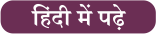SSC CGL Vacancy: Check Vacancies

Click here for best SSC CGL mock tests, video course, live batches, books or eBooks

×

Thank You, Your details have been submitted we will get back to you.Join India largest learning distination

What You Will get ?

•Job Alerts
•Daily Quizes
•Subject-Wise Quizes
•Current Affairs
•previous year question papers
•Doubt Solving session

ORJoin India largest learning distination

What You Will get ?

•Job Alerts
•Daily Quizes
•Subject-Wise Quizes
•Current Affairs
•previous year question papers
•Doubt Solving session

ORJoin India largest learning distination

What You Will get ?

•Job Alerts
•Daily Quizes
•Subject-Wise Quizes
•Current Affairs
•previous year question papers
•Doubt Solving session

Enter the email address associated with your account, and we'll email you an OTP to verify it's you.Join India largest learning distination

What You Will get ?

•Job Alerts
•Daily Quizes
•Subject-Wise Quizes
•Current Affairs
•previous year question papers
•Doubt Solving session

## Enter OTP

Please enter the OTP sent to
/6

Did not recive OTP?

Resend in 60sJoin India largest learning distination

What You Will get ?

•Job Alerts
•Daily Quizes
•Subject-Wise Quizes
•Current Affairs
•previous year question papers
•Doubt Solving sessionJoin India largest learning distination

What You Will get ?

•Job Alerts
•Daily Quizes
•Subject-Wise Quizes
•Current Affairs
•previous year question papers
•Doubt Solving session

## Almost there

+91Join India largest learning distination

What You Will get ?

•Job Alerts
•Daily Quizes
•Subject-Wise Quizes
•Current Affairs
•previous year question papers
•Doubt Solving session

## Enter OTP

Please enter the OTP sent to Edit Number

Did not recive OTP?

Resend 60

## By skipping this step you will not recieve any free content avalaible on adda247, also you will miss onto notification and job alerts

Are you sure you want to skip this step?

## By skipping this step you will not recieve any free content avalaible on adda247, also you will miss onto notification and job alerts

Are you sure you want to skip this step?